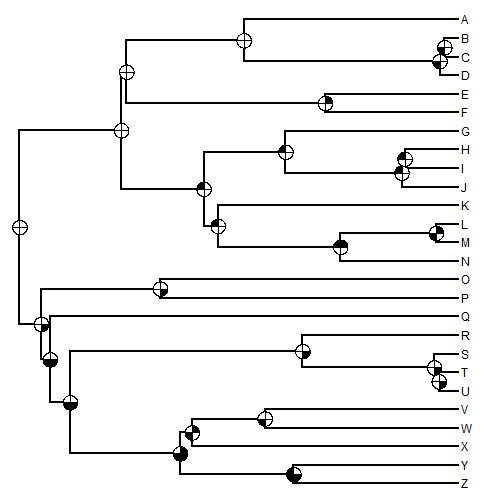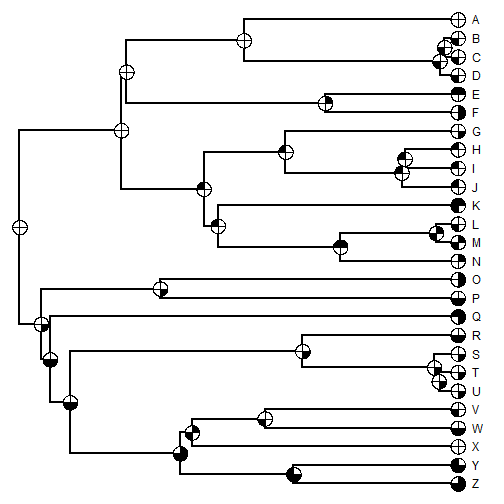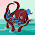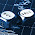## Tuesday, August 12, 2014

### Plotting multiple binary characters at nodes on the tree using pie charts

“I am trying to plot the results of an ancestral state reconstruction of 4 discrete traits onto a single phylogeny. What I would like to do is have a pie on each node split into 4 equal segments, and then have the colour of each segment correspond to the probable trait value at that node (black for present, white for absent, for example).

"Would anyone be able to offer me some guidance as to how to colour the segment based on a dataframe or matrix of trait values rather than the position of the segment in the pie (which is how the piechart legend seems to do it)?”

So far as I can tell, this cannot be done using the standard ape function for plotting pies at nodes, nodelabels; however it is pretty easy to do with a basic understanding of the internal machinations of the nodelabels function. Here is how.

First, let's simulate a tree and presence/absence (binary 0/1) data for four characters:

library(phytools)
## simulate tree
tree<-pbtree(n=26,tip.label=LETTERS[26:1],scale=1)
## transition matrix
Q<-matrix(c(-1,1,1,-1),2,2)
rownames(Q)<-colnames(Q)<-0:1
## simulate character histories
## making "0" the ancestral state
trees<-replicate(sim.history(tree,Q,anc="0"),n=4,simplify=FALSE)
class(trees)<-"multiPhylo"
## pull out the states at nodes
N<-sapply(trees,function(x) as.numeric(getStates(x,"nodes")))
rownames(N)<-1:tree$Nnode+Ntip(tree)  The end product of this simulation is a phylogeny (tree) and a matrix containing 0s and 1s for each node in the tree. This would normally be our input for the next stage, which is our actual plotting exercise tree  ## ## Phylogenetic tree with 26 tips and 25 internal nodes. ## ## Tip labels: ## Z, Y, X, W, V, U, ... ## ## Rooted; includes branch lengths.  N  ## [,1] [,2] [,3] [,4] ## 27 0 0 0 0 ## 28 0 0 0 1 ## 29 0 0 1 1 ## 30 0 0 1 1 ## 31 1 0 1 1 ## 32 0 1 1 1 ## 33 1 0 1 0 ## 34 0 0 1 0 ## 35 0 0 0 1 ## 36 0 0 0 1 ## 37 0 0 0 1 ## 38 0 0 0 1 ## 39 0 0 0 0 ## 40 0 1 0 0 ## 41 0 1 0 0 ## 42 1 1 0 0 ## 43 1 0 1 0 ## 44 0 1 0 0 ## 45 0 1 0 0 ## 46 0 1 0 0 ## 47 0 0 0 0 ## 48 1 0 0 0 ## 49 0 0 0 0 ## 50 0 0 1 0 ## 51 0 0 1 0  OK, here we go. For this, we'll get the help of the package plotrix to plot floating pie charts at all the nodes of the tree: plotTree(tree) ## first part borrowed from nodelabels lastPP<-get("last_plot.phylo",envir=.PlotPhyloEnv) node<-(lastPP$Ntip+1):length(lastPP$xx) X<-lastPP$xx[node]
Y<-lastPP$yy[node] h<-par()$usr
for(i in 1:length(node))
col=c("white","black")[N[i,]+1])It is also straightforward to do the tips as well in the same way:

## preliminaries
## pull out the states at tips
T<-sapply(trees,function(x) as.numeric(getStates(x,"tips")))
rownames(T)<-tree$tip.label ## plot plotTree(tree,offset=1) lastPP<-get("last_plot.phylo",envir=.PlotPhyloEnv) node<-(lastPP$Ntip+1):length(lastPP$xx) X<-lastPP$xx[node]
Y<-lastPP$yy[node] h<-par()$usr
for(i in 1:length(node))
col=c("white","black")[N[i,]+1])
X<-lastPP$xx[1:Ntip(tree)] Y<-lastPP$yy[1:Ntip(tree)]
for(i in 1:Ntip(tree))
col=c("white","black")[T[i,]+1])That's it.

1.2.